## 谁可以从本指南中获益最多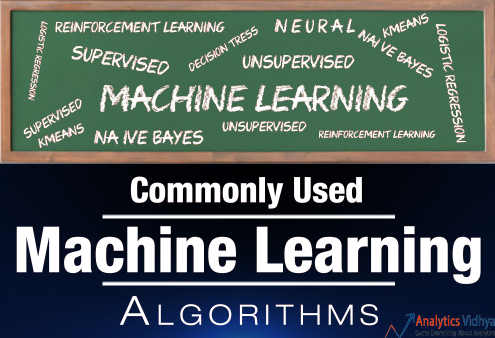## 3类机器学习算法（广义上）

1. 监督学习
工作原理：该算法由一组目标/结果变量（或因变量）组成，该变量将根据给定的一组预测变量（独立变量）进行预测。 使用这些变量集，我们生成一个将输入映射到所需输出的函数。 训练过程继续进行执行，直到模型达到培训数据所需的准确度水平。 监督学习的例子：回归，决策树，随机森林，KNN，逻辑回归等

2. 无监督学习
如何工作：在这个算法中，我们没有任何目标或结果变量来预测/估计。 用于不同群体的群体聚类和用于不同群体的客户进行特定干预。 无监督学习的例子：Apriori算法，K-means。

3. 加强学习
工作原理：使用这种算法，机器受到学习和训练，作出具体决定。 它以这种方式工作：机器暴露在一个环境中，它连续不断地使用试错。 该机器从过去的经验中学习，并尝试捕获最好的知识，以做出准确的业务决策。 加强学习示例：马尔可夫决策过程

1. 线性回归
2. 逻辑回归
3. 决策树
4. SVM
5. 朴素贝叶斯
6. KNN
7. K-Means
8. 随机森林
9. 降维算法

## 1.线性回归

Y-因变量
a - 斜率
X - 自变量
b - 截距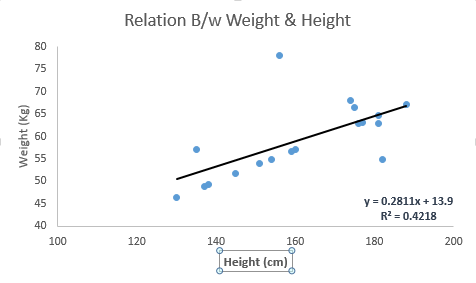#Import Library
#Import other necessary libraries like pandas, numpy...
from sklearn import linear_model
import numpy as np
#Identify feature and response variable(s) and values must be numeric and numpy arrays
# x_train=input_variables_values_training_datasets
x_train=np.random.rand(4,4)
print(x_train)
# y_train=target_variables_values_training_datasets
y_train=np.random.rand(4,4)
print(y_train)
# x_test=input_variables_values_test_datasets
x_test=np.random.rand(4,4)
print(x_test)
# Create linear regression object
linear = linear_model.LinearRegression()
# Train the model using the training sets and check score
linear.fit(x_train, y_train)
linear.score(x_train, y_train)
#Equation coefficient and Intercept
print('Coefficient: \n', linear.coef_)
print('Intercept: \n', linear.intercept_)
#Predict Output
predicted= linear.predict(x_test)
print('predicted:\n',predicted)

[[ 0.98267731  0.23364069  0.35133775  0.92826309]
[ 0.80538991  0.05637806  0.87662175  0.3960776 ]
[ 0.54686738  0.6816495   0.99747716  0.32531085]
[ 0.19189509  0.87105462  0.88158122  0.25056621]]
[[ 0.55541608  0.56859636  0.40616234  0.14683524]
[ 0.09937835  0.63874553  0.92062536  0.32798326]
[ 0.87174236  0.779044    0.79119392  0.06912842]
[ 0.87907434  0.53175367  0.01371655  0.11414196]]
[[ 0.37568516  0.17267374  0.51647046  0.04774661]
[ 0.38573914  0.85335136  0.11647555  0.0758696 ]
[ 0.67559384  0.57535368  0.88579261  0.26278658]
[ 0.13829782  0.28328756  0.51170484  0.04260013]]
Coefficient:
[[ 0.55158868  1.45901817  0.31224322  0.49538173]
[ 0.6995448   0.40804135  0.59938423  0.09084578]
[ 1.79010371  0.21674532  1.60972012 -0.046387  ]
[-0.31562917 -0.53767439 -0.16141312 -0.2154683 ]]
Intercept:
[-0.89705102 -0.50908061 -1.9260686   0.83934127]
predicted:
[[-0.25297601  0.13808785 -0.38696891  0.53426883]
[ 0.63472658  0.18566989 -0.86662193  0.22361739]
[ 0.72181277  0.75309881  0.82170796  0.11715048]
[-0.22656611  0.01383581 -0.79537442  0.55159912]]


R代码

#Load Train and Test datasets
#Identify feature and response variable(s) and values must be numeric and numpy arrays
x_train <- input_variables_values_training_datasets
y_train <- target_variables_values_training_datasets
x_test <- input_variables_values_test_datasets
x <- cbind(x_train,y_train)
# Train the model using the training sets and check score
linear <- lm(y_train ~ ., data = x)
summary(linear)
#Predict Output
predicted= predict(linear,x_test)


## 2. 逻辑回归

odds= p/ (1-p) = probability of event occurrence / probability of not event occurrence ln(odds) = ln(p/(1-p)) logit(p) = ln(p/(1-p)) = b0+b1X1+b2X2+b3X3....+bkXk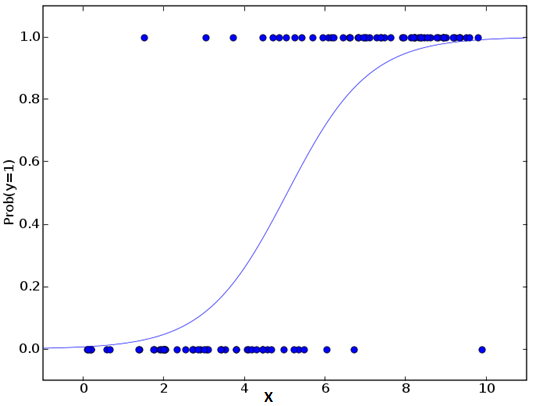Python Code

#Import Library
from sklearn.linear_model import LogisticRegression
#Assumed you have, X (predictor) and Y (target) for training data set and x_test(predictor) of test_dataset
# Create logistic regression object
model = LogisticRegression()
# Train the model using the training sets and check score
model.fit(X, y)
model.score(X, y)
#Equation coefficient and Intercept
print('Coefficient: \n', model.coef_)
print('Intercept: \n', model.intercept_)
#Predict Output
predicted= model.predict(x_test)


R Code

x <- cbind(x_train,y_train)
# Train the model using the training sets and check score
logistic <- glm(y_train ~ ., data = x,family='binomial')
summary(logistic)
#Predict Output
predicted= predict(logistic,x_test)


## 3.决策树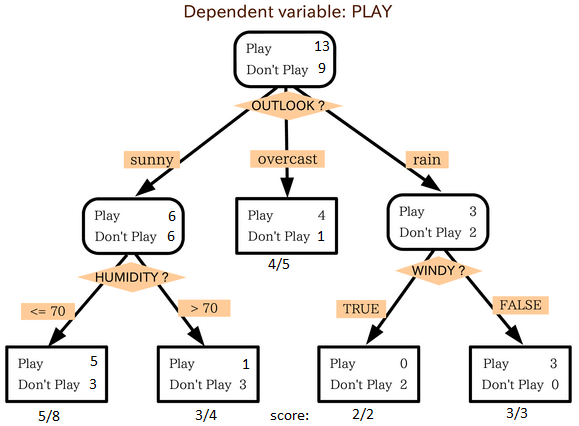Python Code

#Import Library
#Import other necessary libraries like pandas, numpy...
from sklearn import tree
#Assumed you have, X (predictor) and Y (target) for training data set and x_test(predictor) of test_dataset
# Create tree object
model = tree.DecisionTreeClassifier(criterion='gini')
# for classification, here you can change the algorithm as gini or entropy (information gain) by default it is gini
# model = tree.DecisionTreeRegressor() for regression
# Train the model using the training sets and check score
model.fit(X, y)
model.score(X, y)
#Predict Output
predicted= model.predict(x_test)


R Code

library(rpart)
x <- cbind(x_train,y_train)
# grow tree
fit <- rpart(y_train ~ ., data = x,method="class")
summary(fit)
#Predict Output
predicted= predict(fit,x_test)


## 4.SVM(支持向量机)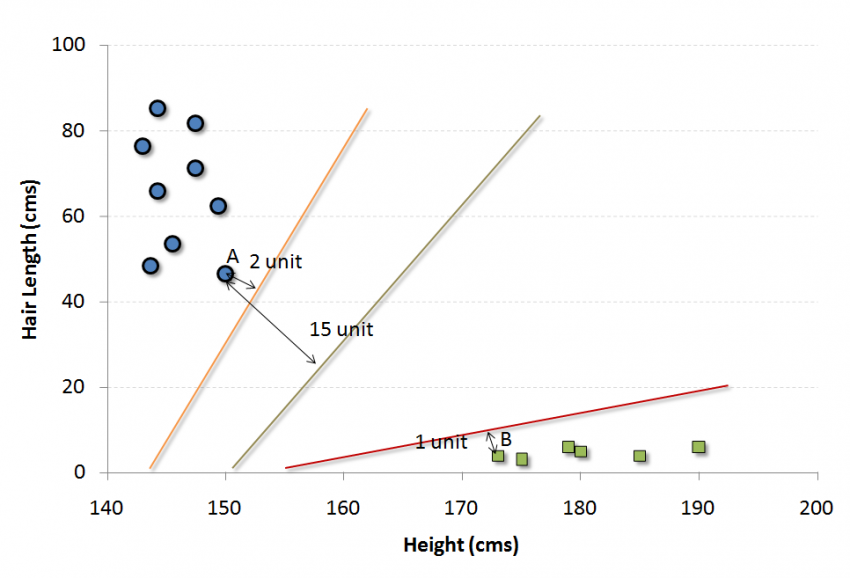Python Code

#Import Library
from sklearn import svm
#Assumed you have, X (predictor) and Y (target) for training data set and x_test(predictor) of test_dataset
# Create SVM classification object
model = svm.svc() # there is various option associated with it, this is simple for classification. You can refer link, for mo# re detail.
# Train the model using the training sets and check score
model.fit(X, y)
model.score(X, y)
#Predict Output
predicted= model.predict(x_test)


R Code

library(e1071)
x <- cbind(x_train,y_train)
# Fitting model
fit <-svm(y_train ~ ., data = x)
summary(fit)
#Predict Output
predicted= predict(fit,x_test)


## 5. 朴素贝叶斯• P（c | x）是在x条件下c发生的概率。
• P（c）是c发生的概率。
• P（x | c）在c条件下x发生的概率。
• P（x）是x发生的概率。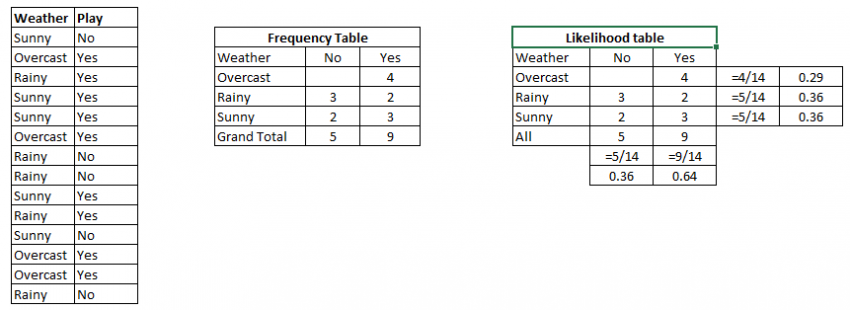Python Code

#Import Library
from sklearn.naive_bayes import GaussianNB
#Assumed you have, X (predictor) and Y (target) for training data set and x_test(predictor) of test_dataset
# Create SVM classification object model = GaussianNB()
# there is other distribution for multinomial classes like Bernoulli Naive Bayes, Refer link
# Train the model using the training sets and check score
model.fit(X, y)
#Predict Output
predicted= model.predict(x_test)


R Code

library(e1071)
x <- cbind(x_train,y_train)
# Fitting model
fit <-naiveBayes(y_train ~ ., data = x)
summary(fit)
#Predict Output
predicted= predict(fit,x_test)


## 6. KNN (K-近邻算法)KNN可以轻松映射到我们的现实生活中。 如果你想了解一个人，你没有任何信息，你可能想知道先去了解他的亲密的朋友和他活动的圈子，从而获得他/她的信息！

• KNN在计算上是昂贵的
• 变量应该被归一化，否则更高的范围变量可以偏移它
• 在进行KNN之前，预处理阶段的工作更像去除离群值、噪声值

Python Code

#Import Library
from sklearn.neighbors import KNeighborsClassifier
#Assumed you have, X (predictor) and Y (target) for training data set and x_test(predictor) of test_dataset
# Create KNeighbors classifier object model
KNeighborsClassifier(n_neighbors=6) # default value for n_neighbors is 5
# Train the model using the training sets and check score
model.fit(X, y)
#Predict Output
predicted= model.predict(x_test)


R Code

library(knn)
x <- cbind(x_train,y_train)
# Fitting model
fit <-knn(y_train ~ ., data = x,k=5)
summary(fit)
#Predict Output
predicted= predict(fit,x_test)


## 7. K-MeansK-means如何形成集群

• （1） 从 n个数据对象任意选择 k 个对象作为初始聚类中心；
• （2） 根据每个聚类对象的均值（中心对象），计算每个对象与这些中心对象的距离；并根据最小距离重新对相应对象进行划分；
• （3） 重新计算每个（有变化）聚类的均值（中心对象）
• （4） 循环（2）到（3）直到每个聚类不再发生变化为止 参考

K-Means例子

1. 随机在图中取K（这里K=2）个种子点。
2. 然后对图中的所有点求到这K个种子点的距离，假如点Pi离种子点Si最近，那么Pi属于Si点群。（上图中，我们可以看到A，B属于上面的种子点，C，D，E属于下面中部的种子点）
3. 接下来，我们要移动种子点到属于他的“点群”的中心。（见图上的第三步）
4. 然后重复第2）和第3）步，直到，种子点没有移动（我们可以看到图中的第四步上面的种子点聚合了A，B，C，下面的种子点聚合了D，E）。参考

K值如何确定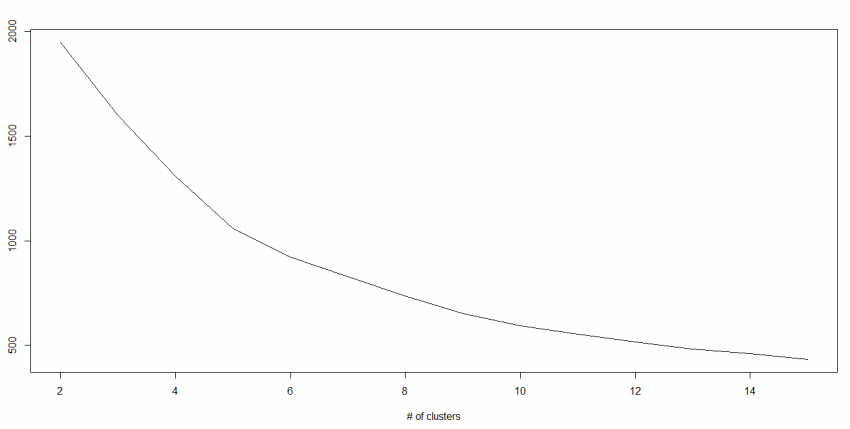Python Code

#Import Library
from sklearn.cluster import KMeans
#Assumed you have, X (attributes) for training data set and x_test(attributes) of test_dataset
# Create KNeighbors classifier object model
k_means = KMeans(n_clusters=3, random_state=0)
# Train the model using the training sets and check score
model.fit(X)
#Predict Output
predicted= model.predict(x_test)


R Code

library(cluster)
fit <- kmeans(X, 3) # 5 cluster solution


## 8. Random Forest（随机树林）## 9. 降维算法

Python Code

#Import Library
from sklearn import decomposition
#Assumed you have training and test data set as train and test
# Create PCA obeject pca= decomposition.PCA(n_components=k) #default value of k =min(n_sample, n_features)
# For Factor analysis
#fa= decomposition.FactorAnalysis()
# Reduced the dimension of training dataset using PCA
train_reduced = pca.fit_transform(train)
#Reduced the dimension of test dataset
test_reduced = pca.transform(test)


R Code

library(stats)
pca <- princomp(train, cor = TRUE)
train_reduced  <- predict(pca,train)
test_reduced  <- predict(pca,test)


Python Code

#Import Library
#Assumed you have, X (predictor) and Y (target) for training data set and x_test(predictor) of test_dataset
# Create Gradient Boosting Classifier object
# Train the model using the training sets and check score
model.fit(X, y)
#Predict Output
predicted= model.predict(x_test)


R Code

library(caret)
x <- cbind(x_train,y_train)
# Fitting model
fitControl <- trainControl( method = "repeatedcv", number = 4, repeats = 4)
fit <- train(y ~ ., data = x, method = "gbm", trControl = fitControl,verbose = FALSE)
predicted= predict(fit,x_test,type= "prob")[,2]